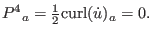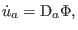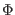### 5.1.2 Acceleration Potential

In an irrotational spacetime, Eq. (46) becomes(75)

It introduces a scalar potential:(76)

whereis the acceleration potential. This scalar potential corresponds to the Newtonian potential. In the irrotational Newtonian model, the linearized acceleration is characterized as the acceleration potential.

Ashkbiz Danehkar
2018-03-26